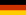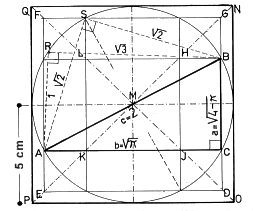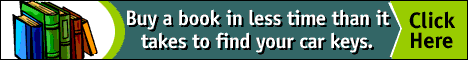Abstract. Engineer Helmut Sander reflects on the impossibility of squaring the circle and the irrational number pi, and provides a geometric construction that approximates the operation.

#A geometrical ensemble to generate the squaring of the circle

Dr.-Ing. Helmut Sander
Hauffstrasse 28
34125 Kassel, Germany

German versionThe purely geometrical squaring of the circle with straightedge and compass is possible only within the tolerance of an approximation. But knowing the value of the irrational number pi of the circle (p = 3,14159265 ...), it is possible to transform it as a line or rather as a shape of a circle or a square. The number of the shape of a circle is pr2 , the number of the shape of the square is (root-pr2 )2 = (r[root-p])2. If the radius of the circle is r = 1, the shape of the circle and the shape of the square DEFG equal p. In the following illustration (Figure 1), the line of the unit is 5 cm. The square of the unit therefore amounts 52 = 25 cm2. The larger square NOPQ goes up to 22 = 4 units (or 102 = 100 cm2). This means that the shapes of circle (= p) and square (= p) are smaller than this larger square.
The shape of the square DEFG = p observes the Pythagorean theorem a2 + b2 = c2 for right triangles, because the two shapes (square DEFG and the surrounding shapes) add up to the square-shape NOPQ. The formula is:

[5(root-p)]2 + [5(root-4 - p)]2 = 25 (p + 4 - p) = 100.The inner circle of the larger square includes the right triangle:

ABC = a:b:c = root(4 - p) : root(p : 2).

The Pythagorean theorem confirms the equation ( 4 - p) + p = 4, and is also applicable to "irrational" right triangles. If you move the point C on its semi-circle between A and B, then there are demonstrated ad infinitum all possible right triangles. The sum of the squared catheti in every case equals 4 (for instance the triangle ABS: 2(root-2)2 = 4; the triangle ABR: 12 + (root-3)2 = 4), because all plane triangles are touching a revolving circle, their forms are represented in the picture of the squaring of the circle. It is a really "cosmological arrangement", because we are able to project the optical vaulted sky on to a planar celestial chart. On the other hand the circle with the radius 1 is the inner circle of a virtual right triangle with the sides 3:4:5. We have a given shape of the square in our design of 100, and in so far we could double this proportion 3:4:5 to 6:8:10 and integrate it into the circle: 62 + 82 = 102 (36 + 64 = 100).

Here we are able to identify a connection to the infinite system of the so-called "primitive Pythagorean triples", which include all natural and all positive real numbers.
The many various imaginable triangles in the circle like ABC can be put together (as a pair; two identical axes) either to symmetrical rectangles or to deltoids (one identical axis), if you superimpose the triangle ABC over the axis AB. Rectangles and deltoids belong to the symmetrical polygons of the circle. The shapes of the polygons, ACBR and ACBS for instance, are nonsymmetrical. Two of the equal rectangles can be put together (by rotation of 90°) to form the arms of a Greek Cross. Its outer corners are the polygonal points of an inscribed symmetrical octagon on the circle with the radius 1. Octagons have a centered symmetry with eight axes. The shape of the inner square of the cross HJKL equals the shape of the slim frame between NOPQ and DEFG. In consequence of this, the shape with the value p is equal to the shape of the larger frame between NOPQ and HJKL. This relationship is valid in every case. Greek Crosses make it easier to make out the relationships of many shapes and numbers within all plane triangles, also between other polygons and concerning the squaring of the circle. The most important aids (for the squaring of the circle) are the Strahlensätze (intercept theorems) and the evident Pythagorean theorem for right triangles. These aids are valid with regards to all plane triangles, including the famous irrational "Fermat triangles" (Pierre de Fermat 1601 - 1665). This hypothesis provided geometrical explanations in correspondence to the theory of numbers. A special case is the centered symmetrical hexagon (Star of King David) with the sides equal to 1, the most simple Fermat-equation 1n + 1n = 2 and the equilateral triangle, the so-called "Eye of God".

Translated by Gert Sperling

REFERENCES
Schröer, Klaus and Klaus Irle . "Ich aber quadriere den Kreis..."
(Münster: Waxmann Verlag, 1998). To order this book from Amazon.de, click here. Or, read the Review by Gert Sperling in the NNJ, vol. 2 no. 1.

RELATED SITES ON THE WORLD WIDE WEB

Dr. Helmut Sander received his diploma in 1938, and studied architecture from 1938 to 1945 at the TH Hannover. He is the technical director of the state building authorities and the University of Kassell, especially for the preservation of historic monuments. He worked in particular on the "Herkules Monument" in Kassel-Wilhelmshoehe. This building was the subject of his doctoral thesis at the University of Berlin in 1981 (ISBN 3-87816-037-2). He has studied the theory of numbers and geometry since 1948. He has published 25 papers concerning Pythagorean theories in scientific periodicals.

 The correct citation for this article is:Helmut Sander, "A Geometrical Ensemble to Generate the Squaring of the Circle", Nexus Network Journal, vol. 2 ( 2000), pp. 83-85. http://www.nexusjournal.com/Sander.htmltop of page

NNJ Homepage

Related Sites on the WWW

Order books!

Research Articles

The Geometer's Angle

Didactics

Book Reviews

Conference and Exhibit Reports